Select Page

Computer Organization

Memory Organization
Interrupt
DMA Controller

# Computer Instructions

Computer instructions are a set of machine language instructions that a particular processor understands and executes. A computer performs tasks according to instructions provided.

## Fields of Computer instructions

There are three basic fields of each instruction in a computer. Let look at the following diagram of 16-bit instruction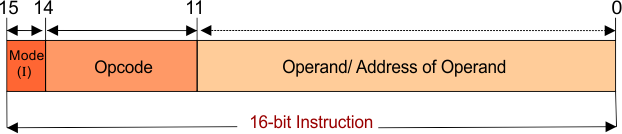I. The Operand is a data. But the operand field may contain actual data or address of that data. Address of data means the location of register or memory where actual data is present.

II. The Opcode field tells the operation which will be performed on data, i.e. +, -, x, <, etc.

III. The Mode field tells about the addressing modes. It is a source to find the operand. It tells whether the operand field contains the actual data or address of memory, address of the register, or address of address, etc. i.e. if Mode bit = 0 then it is direct addressing and if Mode bit =1 then it is an indirect addressing mode. We will cover all of this in detail in addressing modes.

## Computer Instructions Formats

A basic computer has three instruction code formats.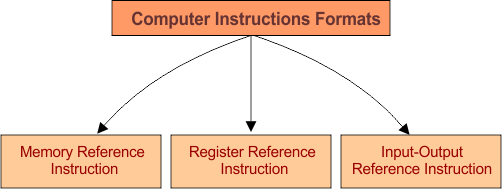### 1. Memory Reference Instruction

The instruction format of memory reference instructions is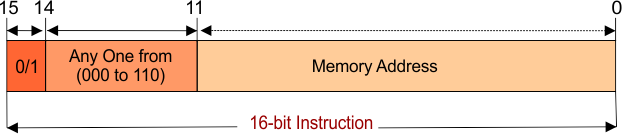• Where the 15th bit which is I. This value of I will be 0, to represent direct addressing mode, or 1 to represent indirect addressing mode.
• 12 to 14 bits represent the Opcode value. Opcode value will be in between 000 to 110. These 7 values (000 to 110) represents the 7 decode operations. Decode operations represented by D0 to D6 as shown in the following diagram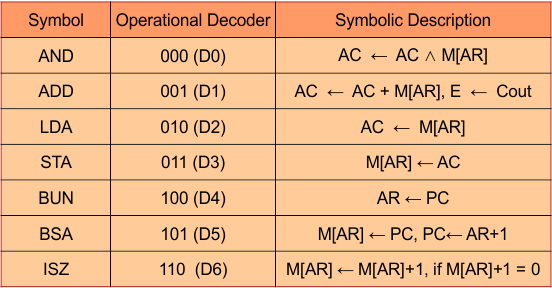when the value of Opcode = 001 (D1) then ADD operation will perform on the operand.

• 0 to 11 bits represent the address of memory location or operand itself.

### 2. Register – Reference Instruction

The instruction format of memory reference instructions is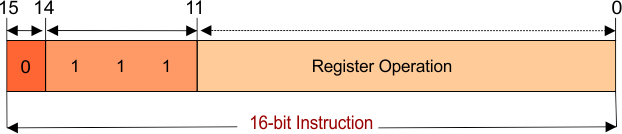• Where the 15th bit which is I will always equal to 0 to represent register addressing mode.
• 12 to 14 bits represent the Opcode value. Opcode value will always equal to 111.
• 0 to 11 bits of operand represents the register operation. Different operations can be performed on the register by setting the value 1 of any location from 0 to 11 bits. So, 12 operations can be performed on registers by setting 1 to different locations (B0 to B11).

For example when the value of operand = 000000000001 then HLT operation will perform on the register.  As shown in the following table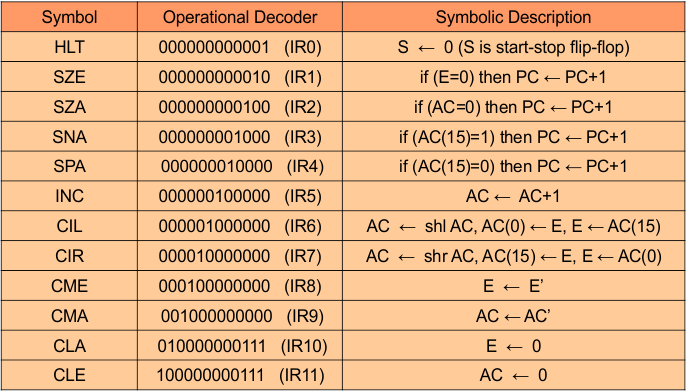### 3. Input-Output Instruction

The instruction format of memory reference instructions is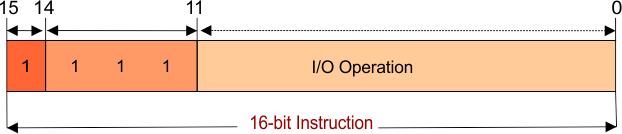• Where the 15th bit which is I will always equal to 1 to represent I/O addressing mode.
• 12 to 14 bits represent the Opcode value. Opcode value will always equal to 111.
• 0 to 5 bits of operand will always be zero and 6-11 bits represents the I/O operation. Different operations can be performed on I/O by setting the value 1 of any location from 6 to 11 bits. So, 6 operations can be performed on I/O by setting 1 to different locations (B6 to B11).

For example when the value of operand = 000001000000  then IOF operation will perform on the register. As shown in the following table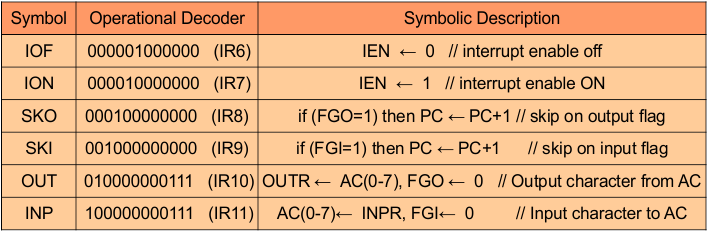## Basic Categories Of Computer Instructions

There are 3 basic categories of instruction in General Computers

• Data transfer instructions
• Data Manipulation instruction
• Program Control Instruction

Note that the above discuss instructions are not specified for a specific CPU like. Intel, AMD, etc. but these instructions are common in all processors.

Help Other’s By Sharing…